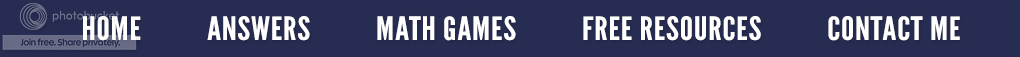Don't Flip!

My college students in remedial math just finished the chapter on fractions.  Talk about mathphobia.  Dividing fractions was the most confusing for them because it requires finding the reciprocal of the second fraction, changing the division sign to a multiplication sign, and then multiplying the numerator times the numerator and the denominator times the denominator.

Let me introduce a new method entitled Just Cross.

1)      First and foremost, you must understand what division is.  The statement 8 ÷ 4 means 8 divided into 4 equal sets, OR how many fours are in eight, OR how many times can we subtract 4 from 8?  (Yes, division is repeated subtraction.)
2)      Let me explain this using a hands-on visual.  Let’s assume the fraction problem is:

The question being asked is, How many ¼’s are in ½?”First, fold a piece of paper in half.  The figure on the left represents ½.  Next, fold the same sheet of paper in half again to make fourths as seen in the illustration on the right.  When you unfold the paper, you will notice a total of four sections.  So answering the original question: How many ¼’s are in ½”, you can see that the half sheet of paper contains two parts; therefore:

3)      Using the same example, to work the problem, the fraction 1/4 would have to be flipped to 4/1  nd then 1/2 would have to multiplied by 4/1 to get the correct answer of 2.  That is why the division of fractions requires that the second fraction be inverted and the division sign be changed to a multiplication sign.

Let’s use the same fraction problem, but let’s utilize a different method entitled Just Cross.

1)  Cross your arms as a hands-on way of remembering the process.
2)  Now multiply the denominator of 4 by 1 and the denominator of 2 by1 as seen below.  (We do nothing with the denominators.)  Notice we always start on the left side and then we go to the right side.  I often tell my students it is, "Left, right; left, right"  as if we are marching.  If it is done the opposite way, the answer will be incorrect.

3)      Now simply divide 4 by 2 to get the answer of 2.

No flipping; no reciprocal, no changing the division sign to a multiplication sign.
Just Cross and divide.  Amazingly, it works every time.

I have a resource that features different ways to teach fractions using hands-on strategies similar to the one above.  Just go to Fractions for the Confused and Bewildered.